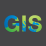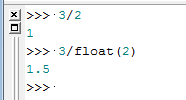# Dividing Integers to create field type :DOUBLE output using Python

3053
4
Jump to solution
08-14-2015 03:06 AMOccasional Contributor

I extracted point data from a raster, using the GRID_CODE value from the raster data. This creates an integer field in the vector output. I needed to use this output in a division calculation but the answer had to be as a field type DOUBLE (i.e. I needed a decimal value). When doing this in a model using the CALCULATE FIELD tool, with the PYTHON syntax option, it would only produce an Integer value. Apparently this is a PYTHON limitation, judging by the Google search I ran on this issue. I did a work-around by creating a temporary DOUBLE field, copied the GRID_CODE value to it and then ran the CALCULATE FIELD division operation. I then deleted the temporary field. This works ok but is very cumbersome. Is there a smart way to set the PYTHON code so that it will divide an integer and produce a decimal output?

The CALCULATE FIELD tool, with the VB syntax option, works fine, but VB is not supported in ArcGIS Pro 64Bit so I can foresee problems in using VB in the near future?

Thanks very much.

Tags (4)
1 Solution

Accepted SolutionsbyMVP Esteemed Contributor

!GRIDE_CODE! /10.0

4 RepliesbyEsri Esteemed Contributor

Hi Mark,

If you are trying to calculate a decimal to a Long/Short Integer field, this will not work.  The field type will have to be Double/Float.  If you simply are calculating the integer value in python, you can convert the value to a float before doing the division.  Ex:Occasional Contributor

Hi Jake,

Thanks for your reply. The raster values were decimetres contained in an integer type field (GRID_CODE). I needed to convert these decimetre values to metres by dividing by 10. When I used the python formula !GRIDE_CODE! / 10, the result was a integer type, i.e. 53 became 5, instead of the 5.3 result I required. I had a look at the code snippet you included, but have not been able to make it work in the FIELD CALCULATOR. How would it look like in the Field Calculator? I am afraid my Python skills are very thin as I am just starting down that road! Thanks again.byMVP Esteemed Contributor

!GRIDE_CODE! /10.0Occasional Contributor

Hi Dan,

tch! So simple :-(.  Thanks so much, you have halved the complexity of my model.